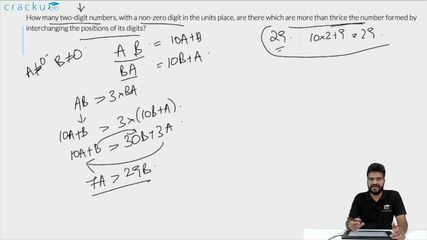Question 72

# How many two-digit numbers, with a non-zero digit in the units place, are there which are more than thrice the number formed by interchanging the positions of its digits?

Solution

Let 'ab' be the two digit number. Where b $$\neq$$ 0.

We will get number 'ba' after interchanging its digit.

It is given that 10a+b > 3*(10b + a)

7a > 29b

If b = 1, then a = {5, 6, 7, 8, 9}

If b = 2, then a = {9}

If b = 3, then no value of 'a' is possible. Hence, we can say that there are a total of 6 such numbers.

### View Video Solution